#EXPONENTIAL FUNCTIONSThe exponential function f with base a is denoted by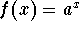, where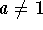, and x is any real number. The function value will be positive because a positive base raised to any power is positive. This means that the graph of the exponential functionwill be located in quadrants I and II.

For example, if the base is 2 and x = 4, the function value f(4) will equal 16. A corresponding point on the graph of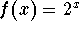would be (4, 16).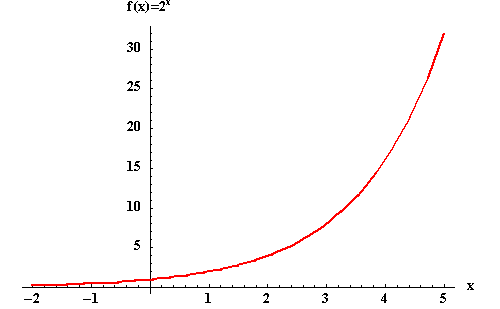For x >0, a>0 , and, we have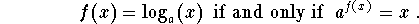Since x > 0, the graph of the above function will be in quadrants I and IV.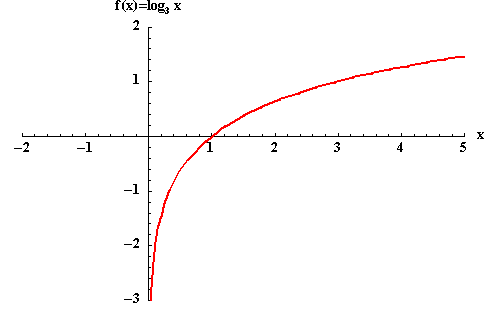• The exponential equation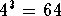could be written in terms of a logarithmic equation as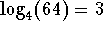.
• The exponential equation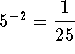can be written as the logarithmic equation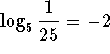.
• Since logarithms are nothing more than exponents, you can use the rules of exponents with logarithms.
• Logarithmic functions are the inverse of exponential functions. For example if (4, 16) is a point on the graph of an exponential function, then (16, 4) would be the corresponding point on the graph of the inverse logarithmic function.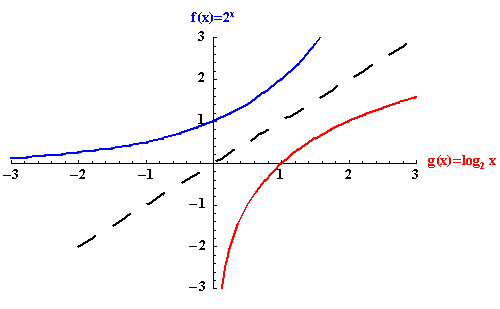• The two most common logarithms are called common logarithms and natural logarithms. Common logarithms have a base of 10, and natural logarithms have a base of e.

If you are interested in reviewing any of the following topics, click the appropriate item:

• The properties of logarithms along with examples and problems, click on Properties
• The graphs of logarithms, with examples and problems, click on Graphs of Logarithms
• Change of base with respect to logarithms with examples and problems, click on Change of base
• The three rules of logarithms, with examples and problems, click on Rules of Logarithms
• Solving exponential and logarithms equations with examples and problems, click on Solving Equations
• Solving word problems involving exponential and logarithms functions with examples and problems, click on Solving Word Problems

•[Exponential Rules] [Logarithms]

[Algebra] [Trigonometry ] [Complex Variables]S.O.S MATHematics home page

Do you need more help? Please post your question on our S.O.S. Mathematics CyberBoard.Author: Nancy Marcus